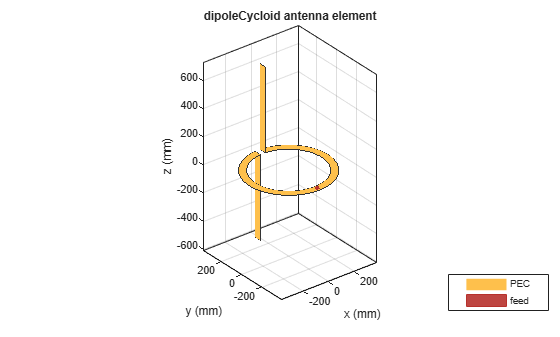# dipoleCycloid

Create cycloid dipole antenna

## Description

The `dipoleCycloid` object is a half-wavelength cycloid dipole antenna. For the default cycloid dipole, the feed point is on the loop section. The default length is for an operating frequency of 48 MHz.The width of the dipole is related to the circular cross-section by the equation

`$w=2d=4r$`

, where:

• d is the diameter of equivalent cylindrical pole

• r is the radius of equivalent cylindrical pole

For a given cylinder radius, use the `cylinder2strip` utility function to calculate the equivalent width.

## Creation

### Syntax

``dc = dipoleCycloid``
``dc = dipoleCycloid(Name,Value)``

### Description

example

``` `dc = dipoleCycloid` creates a half-wavelength cycloid dipole antenna oriented along Z-axis.```

example

``` `dc = dipoleCycloid(Name,Value)` creates a half-wavelength cycloid dipole antenna, with additional properties specified by one or more name-value pair arguments. `Name` is the property name and `Value` is the corresponding value. You can specify several name-value pair arguments in any order as `Name1`, `Value1`, `...`, `NameN`, `ValueN`. Properties not specified retain their default values.```

## Properties

expand all

Dipole length along z-axis, specified as a scalar in meters. By default, the length is for an operating frequency of 48 MHz.

Example: `'Length',0.9`

Data Types: `double`

Dipole width, specified as a scalar in meters.

Example: `'Width',0.09`

Data Types: `double`

Circular loop radius in X-Y plane, specified as a scalar in meters.

Example: `'LoopRadius',0.500`

Data Types: `double`

Gap of loop in X-Y plane, specified as a scalar in meters.

Example: `'Gap',0.006`

Data Types: `double`

Lumped elements added to the antenna feed, specified as the comma-separated pair consisting of `'Load'` and a lumped element object handle. For more information, see `lumpedElement`.

Example: `'Load',lumpedelement`. `lumpedelement` is the object handle for the load created using `lumpedElement`.

Example: ```dc.Load = lumpedElement('Impedance',75)```

Tilt angle of the antenna, specified as a scalar or vector with each element unit in degrees. For more information, see Rotate Antennas and Arrays.

Example: `'Tilt',90`

Example: `ant.Tilt = 90`

Example: `'Tilt',[90 90]`,`'TiltAxis',[0 1 0;0 1 1]` tilts the antenna at 90 degrees about the two axes, defined by vectors.

### Note

The `wireStack` antenna object only accepts the dot method to change its properties.

Data Types: `double`

Tilt axis of the antenna, specified as:

• Three-element vectors of Cartesian coordinates in meters. In this case, each vector starts at the origin and lies along the specified points on the X-, Y-, and Z-axes.

• Two points in space, each specified as three-element vectors of Cartesian coordinates. In this case, the antenna rotates around the line joining the two points in space.

• A string input describing simple rotations around one of the principal axes, 'X', 'Y', or 'Z'.

Example: `'TiltAxis',[0 1 0]`

Example: `'TiltAxis',[0 0 0;0 1 0]`

Example: `ant.TiltAxis = 'Z'`

### Note

The `wireStack` antenna object only accepts the dot method to change its properties.

## Object Functions

 `show` Display antenna or array structure; display shape as filled patch `info` Display information about antenna or array `axialRatio` Axial ratio of antenna `beamwidth` Beamwidth of antenna `charge` Charge distribution on metal or dielectric antenna or array surface `current` Current distribution on metal or dielectric antenna or array surface `design` Design prototype antenna or arrays for resonance at specified frequency `EHfields` Electric and magnetic fields of antennas; Embedded electric and magnetic fields of antenna element in arrays `impedance` Input impedance of antenna; scan impedance of array `mesh` Mesh properties of metal or dielectric antenna or array structure `meshconfig` Change mesh mode of antenna structure `pattern` Radiation pattern and phase of antenna or array; Embedded pattern of antenna element in array `patternAzimuth` Azimuth pattern of antenna or array `patternElevation` Elevation pattern of antenna or array `returnLoss` Return loss of antenna; scan return loss of array `sparameters` S-parameter object `vswr` Voltage standing wave ratio of antenna

## Examples

collapse all

Create a default cycloid dipole antenna using the dipoleCycloid object and view it.

`dc = dipoleCycloid`
```dc = dipoleCycloid with properties: Length: 1.2200 Width: 0.0508 LoopRadius: 0.3100 Gap: 0.0400 Tilt: 0 TiltAxis: [1 0 0] Load: [1x1 lumpedElement] ```
`show(dc)`Calculate the impedance of a cycloid dipole of width, 0.05 m, over a frequency span of 50 MHz - 100 MHz.

```d = dipoleCycloid('Width',0.05); impedance(d,linspace(50e6,100e6,51))```Plot the radiation pattern of a cycloid dipole of width,0.05 m, at a frequency of 48 MHz.

`d = dipoleCycloid('Width',0.05)`
```d = dipoleCycloid with properties: Length: 1.2200 Width: 0.0500 LoopRadius: 0.3100 Gap: 0.0400 Tilt: 0 TiltAxis: [1 0 0] Load: [1x1 lumpedElement] ```
`pattern(d,48e6)`Balanis, C.A. Antenna Theory: Analysis and Design. 3rd Ed. New York: Wiley, 2005.

 Volakis, John. Antenna Engineering Handbook. 4th Ed. New York: McGraw-Hill, 2007.